# GSEB Solutions Class 9 Maths Chapter 5 Introduction to Euclid’s Geometry Ex 5.1

Gujarat Board GSEB Solutions Class 9 Maths Chapter 5 Introduction to Euclid’s Geometry Ex 5.1 Textbook Questions and Answers.

## Gujarat Board Textbook Solutions Class 9 Maths Chapter 5 Introduction to Euclid’s Geometry Ex 5.1

Question 1.
Which of the following statements are true and which are false? Give reasons for your answers.

1. Only one line can pass through a single point.
2. There is an infinite number of lines that pass through two distinct points.
3. A terminated line can be produced indefinitely on both sides.
4. If two circles are equal, then their radii are equal.
5. In figure, if AB = PQ and PQ = XY, then AB = XY.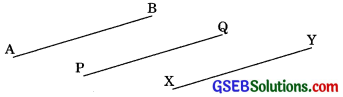Solution:

1. False, through a single point, an infinite number of lines can pass. In the following figure, we can see that there are infinite numbers of lines passing through a single point O.
2.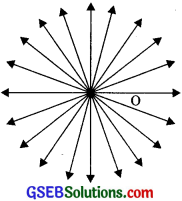3. False, since through two points, one and only one line can pass. We can see in the following figure only one line passes through the two distinct points A and B.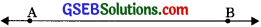4. True, a terminated line can be produced indefinitely on both sides. Let AB is a terminated line. We can see that it can be extended on both sides.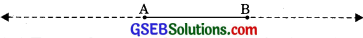5. True, if two circles are equal, then their center and circumferences will coincide and therefore their radii will also be equal.
6. True, it is given that AB and XY are two terminated lines and both are equal to a third line PQ. Euclid’s axiom 1 says that things which are equal to the same thing are equal to one another.

Question 2.
Give a definition for each of the following terms. Are there other terms that need to be defined first? What are they, and how might you define them?

1. Parallel lines
2. Perpendicular lines
3. Line segment
4. The radius of a circle
5. Square

Solution:
1. Parallel lines: Those lines which do not intersect each other are called parallel lines. or if the perpendicular distance between two lines remains constant then these lines are said to be parallel. Example, AB || CD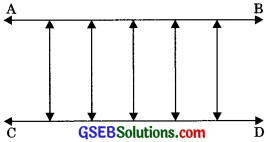To define parallel lines, we must know about points, lines, the distance between the lines, and the point of intersection.

2. Perpendicular lines: If two lines intersect each other at 90°, then such lines are called perpendicular lines.
Example: CD ⊥ AB
or AB ⊥ CD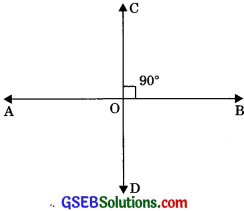Here we first need to define line and angle.
3. Line segment: A straight line drawn from any point to any other point.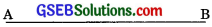Before defining a line segment we should know the point and straight line.
4.  The radius of a circle: Distance between the center of a circle to any point lying on the circumference of the circle.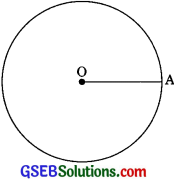Point and circle should be defined before defining the radius of a circle.
5. Square: A square is a quadrilateral having all sides of equal length and all angles of the same measure i.e., 90°.
Or
A rectangle whose one angle is 90°.
Example: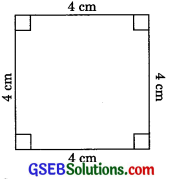To define a square, we must know about quadrilateral, side and angle.

Question 3.
Consider two ‘postulates’ given below:
1. Given any two distinct points A and B, there exists a third point C which is in between A and B.
2. There exist at least three points that are not on the same line.
Do these postulates contain any undefined terms? Are these postulates consistent? Do they follow from Euclid’s postulates? Explain.
Solution:
1. Yes these postulates contain two undefined terms point and line.
2. Yes, these postulates are consistent because they led with two distinct situations
(a) given two points A and B there exist a point C lying on the line in between them
(b) given two points A and B, we can take another point C which does not lie on the line which passes through A and B.
Therefore these postulates do not follow, from Euclid’s postulates however, they follow from Axiom 5.1.Question 4.
If a point C lies between two points A and B such that AC = BC, then prove that AC = $$\frac {1}{2}$$ AB. Explain by drawing the figure.
Solution:
AC = BC (Given)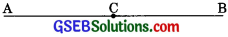AC + AC = BC + AC (Equals are added to equals)
⇒ 2AC = AB (BC + AC coincides with AB)
⇒ AC = $$\frac {1}{2}$$ AB (halves of equals are equal)

Question 5.
In Question 4, point C is called a mid-point of line segment AB. Prove that every line segment has one and only one mid-point.
Solution:
Let line segment has two mid-points.
If C is mid-point between A and B, then
AC = $$\frac {1}{2}$$ AB …….(1) (Proved in Question 4)
If D is mid-point (supposed), then
AD = $$\frac {1}{2}$$ AB …….(2)
From eqn. (1) and (2),
This result shows that C and D coincide, hence every line segment has one and only one mid-point.Question 6.
In figure, if AC = BD, then prove that AB = CD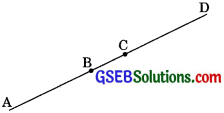Solution:
AC = BD ……(1) (given)
AC = AB + BC …..(2) (point B lies between A and C)
BD – BC + CD …….(3)
(point C lies between B and D) Substituting the value of eqn. (2) and (3) in eqn. (1), we get
AB + BC = BC + CD
⇒ AB = CD (Subtracting equals from equals)

Question 7.
Why is Axiom 5, in the list of Euclid’s axioms, considered a ‘universal truth’? (Note that the question is not about the fifth postulate).
Solution:
Because this is true for anything in any part of the world. Therefore it is a universal truth.